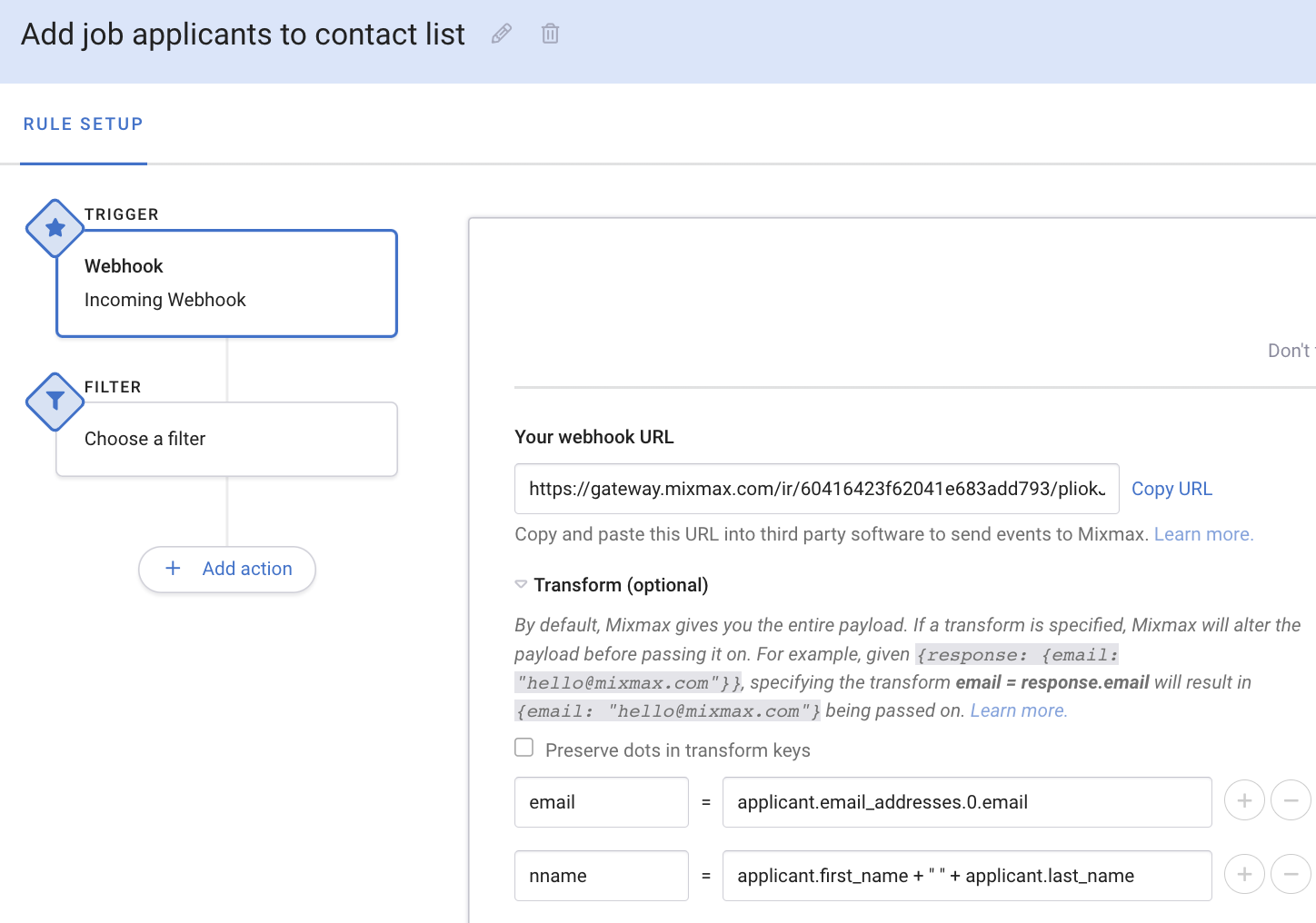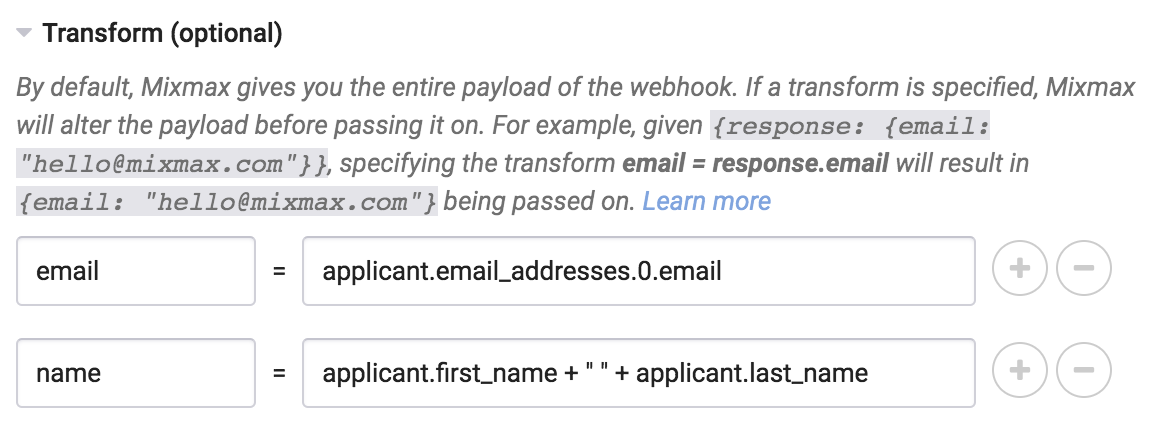# Mixmax Webhook Transform Language (Advanced)

In this article, we discuss how to use the  Mixmax Webhook Transform Language feature of Mixmax Rules.By default, when using the  Incoming webhook Mixmax Rule, Mixmax will pass on the entire payload (the data, typically in JSON format) of the event to the rule "action" (under Then). This is often not desired. For example, if you're receiving a webhook from a third party system with the following payload:

```{
"applicant": {
"first_name": "Jane",
"last_name": "Candidate"
"type": "work",
"email": "jane@example.com"
]
}
}
```

and you're using a Mixmax Rule action such as add to sequence that requires `email` and `name` properties at the root-level, you need to set a transform to convert the webhook to:

```{
"name": "Jane Candidate",
"email": "jane@example.com"
}
```

In order to do this, you'll need to specify the following webhook transforms:Transform "Preserve Dots" checked Description Before After a = b.c n/a Returns a nested property ` {"b" : {"c": 1}} ` ` {"a": 1} ` a = b.0 n/a Returns the Nth element of an array ` {"b": } ` ` {"a": 1} ` a.b.0 = z no Sets a nested property ` {"z": 1} ` ` {"a": {"b": [1}} ` a.b.0 = z yes Sets a property, ignoring dots `{"z": 1}` `{"a.b.0": 1}` a = b[index] n/a Returns the Nth element of an array, using another property as the index ` {"b": [10,11], "index": 1} ` ` {"a": 11} ` a = b.c + d.b n/a Concatenates (joins) two strings `{"b": {"c": "x", "d": "y"}}` `{"a": "xy"}` a = b.c + d.b n/a Adds two numbers (only if inputs are numbers) `{"b": {"c": 1, d: 2}}` `{"a": 3}` a = "string literal" n/a Sets a string literal (useful for "hardcoding" properties when posting to a webhook) ` {"b": 1} ` ` {"a": "string literal"} ` a = b.c + "-" + b.d n/a Concatenates with a string literal ` {"b": {"c": "x", "d": "y"}} ` ` {"a": "x-y"} ` c = . n/a Returns the root object ` {"a": 1, "b": 2} ` ` {"c": {"a": 1, "b": 2}}